## 7-2 Time-domain: PDF: ACF

In this section, we shall introduce the auto-correlation function (ACF) for pitch tracking. This is a time-domain method which estimates the similarity between a frame $s(i), i = 0, \dots, n-1$, and its delayed version via the auto-correlation function: $$acf(\tau)=\sum_{i=0}^{n-1-\tau}s(i)s(i+\tau)$$

where $\tau$ is the time lag in terms of sample points. The value of $\tau$ that maximizes $acf(\tau)$ over a specified range is selected as the pitch period in sample points. The following figure demonstrates the operation of ACF: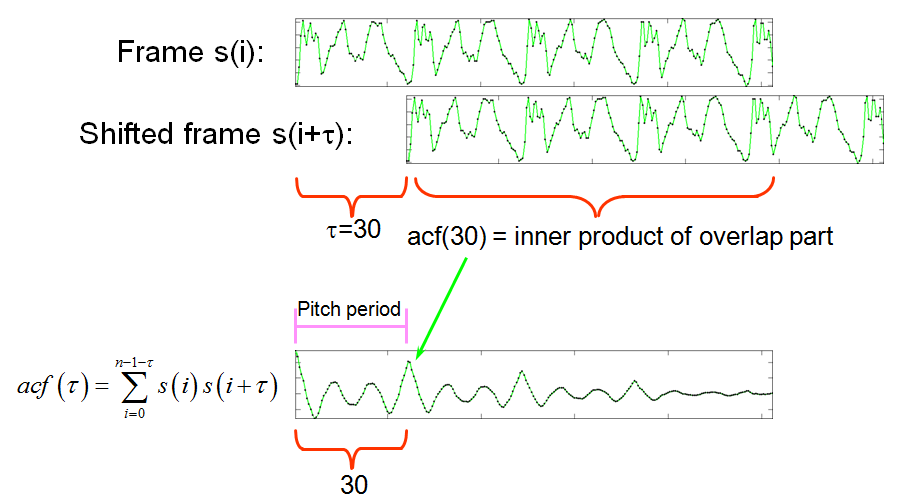In other words, we shift the delayed version n times and compute the inner product of the overlapped parts to obtain n values of ACF.

Take my utterance sunday.wav for example. If we take a frame of 512 points starting from the 9000th point, which corresponds to the vowel part of "day", the ACF result is the following example:

Example 1: frame2acf01.mwaveFile='sunday.wav'; au=myAudioRead(waveFile); index1=9000; frameSize=512; index2=index1+frameSize-1; frame=au.signal(index1:index2); opt=frame2pdf('defaultOpt'); opt.pdf='acf'; opt.maxShift=length(frame); opt.method=1; acf=frame2pdf(frame, opt); subplot(3,1,1); plot(au.signal); line(index1*[1 1], [-1 1], 'color', 'r'); line(index2*[1 1], [-1 1], 'color', 'r'); subplot(3,1,2); plot(frame); subplot(3,1,3); plot(acf);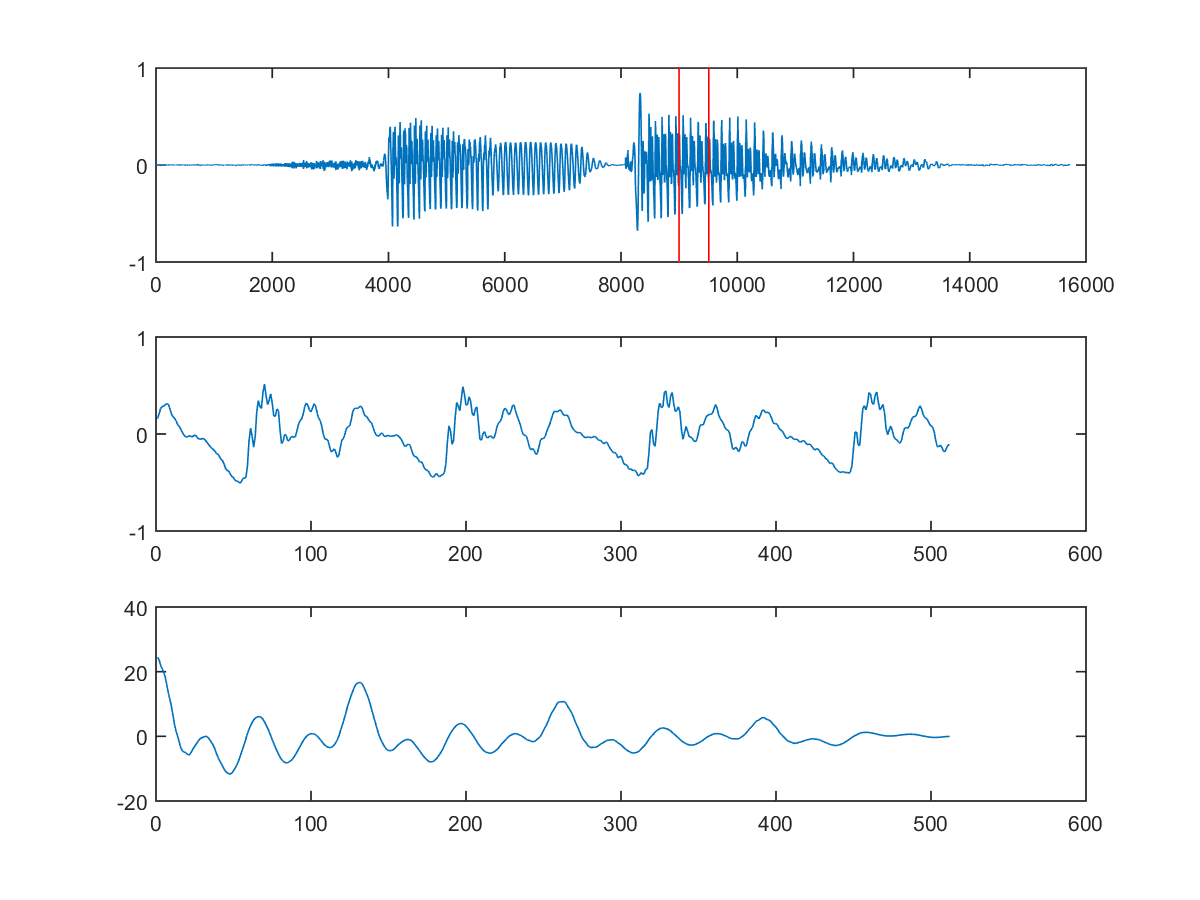The maximum of ACF occurs at the first point, which is obviously not what we want. If we set the values around the first maximum to be $-\infty$, we can identify the second maximum located at index 131 and the corresponding pitch is fs/(131-1) = 16000/130 = 123.08 Hz, or 46.94 semitones.

Hint
In the above computation, we need to divide fs by 131 minus 1 since 131 is a 1-based index in MATLAB.

The point of ACF at index 131 is referred as the pitch point for ACF. In order to identify the pitch point of ACF automatically, we can simply set the first few points of ACF to be $-\infty$ and then find the maximal point of ACF. Please refer to the following example.

Example 2: frame2acfPitchPoint01.mwaveFile='sunday.wav'; au=myAudioRead(waveFile); y=au.signal; fs=au.fs; index1=9000; frameSize=512; index2=index1+frameSize-1; frame=y(index1:index2); maxShift=length(frame); method=1; acf=frame2acf(frame, maxShift, method); acf2=acf; maxFreq=1000; n1=floor(fs/maxFreq+1); acf2(1:n1)=-inf; minFreq=40; n2=ceil(fs/minFreq+1); acf2(n2:end)=-inf; [maxValue, maxIndex]=max(acf2); fprintf('Pitch = %f Hz = %f semitone\n', fs/(maxIndex-1), freq2pitch(fs/(maxIndex-1))); subplot(2,1,1); plot(frame, '.-'); title('Input frame'); subplot(2,1,2); xVec=1:length(acf); plot(xVec, acf, '.-', xVec, acf2, 'm.-', maxIndex, maxValue, 'ksquare'); axisLimit=axis; line(n1*[1 1]+0.5, axisLimit(3:4), 'color', 'r'); line(n2*[1 1]-0.5, axisLimit(3:4), 'color', 'r'); title(sprintf('ACF vector (method = %d)', method)); legend('Original ACF', 'Truncated ACF', 'ACF pitch point');Pitch = 123.076923 Hz = 46.944681 semitone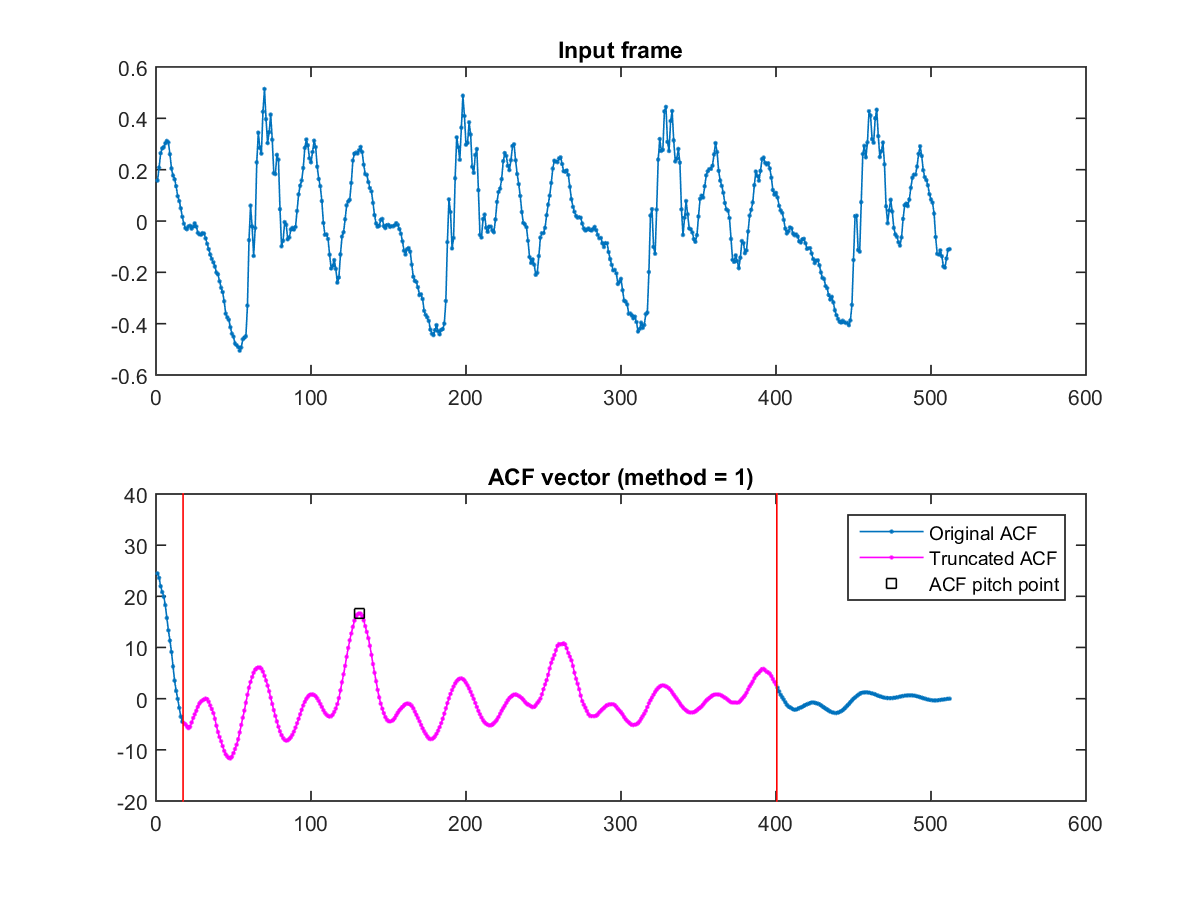In the above figure, the first plot if the frame, the second plot is the original ACF and the modified ACF. The red circle is the maximal point of the modified ACF, which is also the correct pitch point.

The modified ACF is obtained by setting ACF to $-\infty$ at some regions. More specifically, suppose human's pitch is within the range of [40, 1000] (Hz), then we have the following inequality: $$40 \leq \frac{fs}{pp-1} \leq 1000$$ This leads to the range of $pp$ (pitch point): $$\frac{fs}{1000}+1 \leq pp \leq \frac{fs}{40}+1$$

By using the range of $pp$, we can perform pitch tracking on a stream of audio signals, as shown in the next example.

Example 3: wave2pitchByAcf01.mwaveFile='soo.wav'; au=myAudioRead(waveFile); y=au.signal; fs=au.fs; y=y-mean(y); frameDuration=32; % Duration (in ms) of a frame frameSize=round(frameDuration*fs/1000); overlap=0; maxShift=frameSize; maxFreq=1000; minFreq=40; n1=round(fs/maxFreq); % pdf(1:n1) will not be used n2=round(fs/minFreq); % pdf(n2:end) will not be used frameMat=enframe(y, frameSize, overlap); frameNum=size(frameMat, 2); volume=frame2volume(frameMat); volumeTh=max(volume)/10; pitch=0*volume; for i=1:frameNum % fprintf('%d/%d\n', i, frameNum); frame=frameMat(:, i); pdf=frame2acf(frame, maxShift, 1); pdf(1:n1)=-inf; pdf(n2:end)=-inf; [maxValue, maxIndex]=max(pdf); freq=fs/(maxIndex-1); pitch(i)=freq2pitch(freq); end frameTime=frame2sampleIndex(1:frameNum, frameSize, overlap)/fs; subplot(3,1,1); plot((1:length(y))/fs, y); set(gca, 'xlim', [-inf inf]); title('Waveform'); subplot(3,1,2); plot(frameTime, volume, '.-'); set(gca, 'xlim', [-inf inf]); line([0, length(y)/fs], volumeTh*[1, 1], 'color', 'r'); title('Volume'); subplot(3,1,3); pitch2=pitch; pitch2(volume<volumeTh)=nan; % Volume-thresholded pitch plot(frameTime, pitch, frameTime, pitch2, '.-r'); axis tight; xlabel('Time (second)'); ylabel('Semitone'); title('Original pitch (blue) and volume-thresholded pitch (red)'); frameRate=fs/(frameSize-overlap); % No. of pitch points per sec pv.fs=16000; pv.nbits=16; % Specs for saving the synthesized pitch pv.signal=pv2wave(pitch2, frameRate, pv.fs); % Convert pitch to wave pv.amplitudeNormalized=1; myAudioWrite(pv, 'sooPitchByAcf.wav'); % Save the pv as a wav file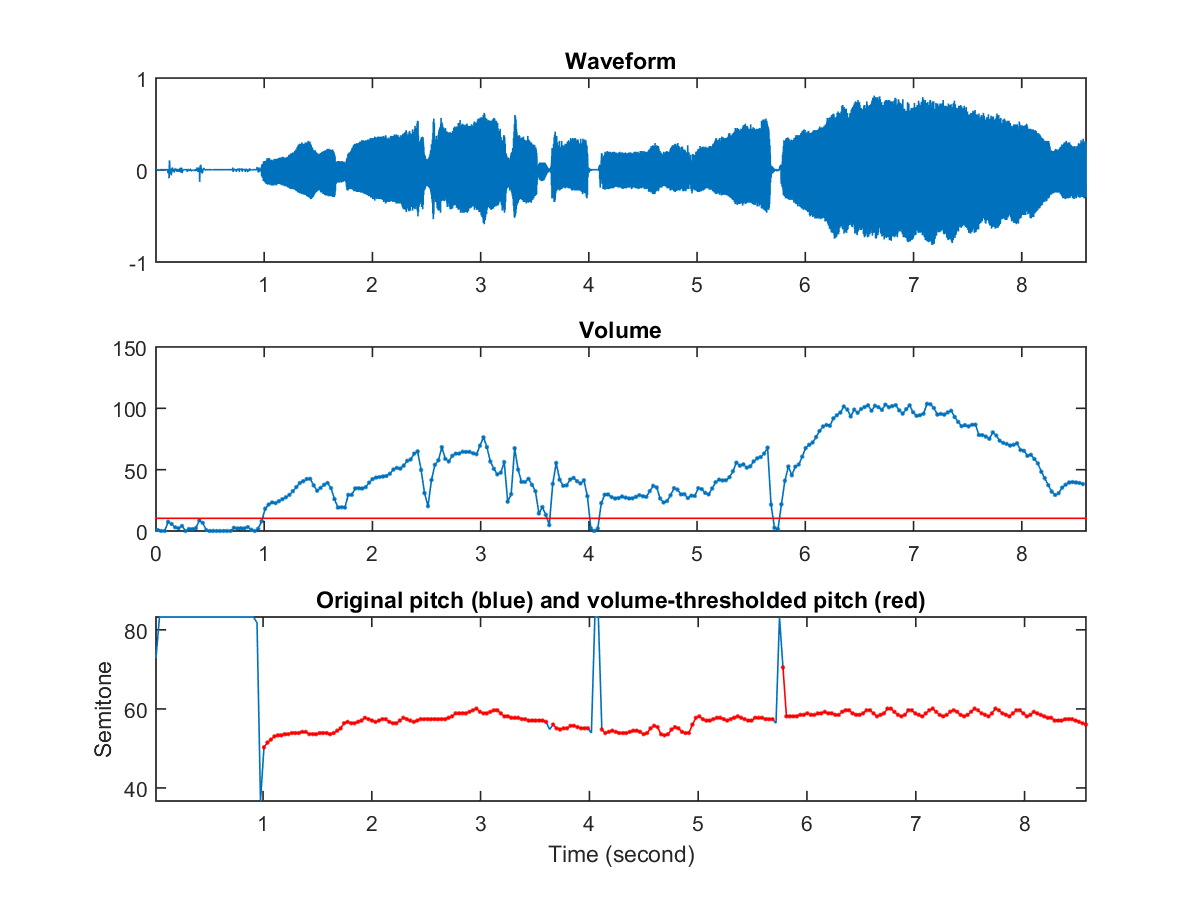In the above example, we use simple volume thresholding with a threshold equal to one eighth of the maximum volume. That is, if a frame has a volume less than 1/8 of the maximum volume, then its pitch is set to zero. From the last plot of the above figure, it is obvious that the values of the pitch curve is mostly correct except for several erroneous points which deviate from the presumably smooth pitch curve. This discontinuous points will cause squeaky sounds during the playback of the pitch curve. We usually apply a smoothing operator (such as median filters) on the pitch curve as a post-process to eliminate erroneous points. Without using the smoothing operator, the results are shown in the following links:

Since the above script is useful for pitch tracking, we have packed it into a function "pitchTrackBasic.m", with an example shown next:

Example 4: ptByAcf01.mauFile='soo.wav'; opt=pitchTrackBasic('defaultOpt'); showPlot=1; pitch=pitchTrackBasic(auFile, opt, showPlot); au=myAudioRead(auFile); frameSize=(opt.frameDuration*au.fs)/1000; hopSize=(opt.hopDuration*au.fs)/1000; frameRate=au.fs/hopSize; % No. of pitch points per sec pv.fs=16000; pv.nbits=16; % Specs for saving the synthesized pitch pv.signal=pv2wave(pitch, frameRate, pv.fs); % Convert pitch to wave pv.amplitudeNormalized=1; myAudioWrite(pv, 'sooPitchByAcf.wav'); % Save the pv as a wav fileError in running ptByAcf01! (Logged to scriptError.log)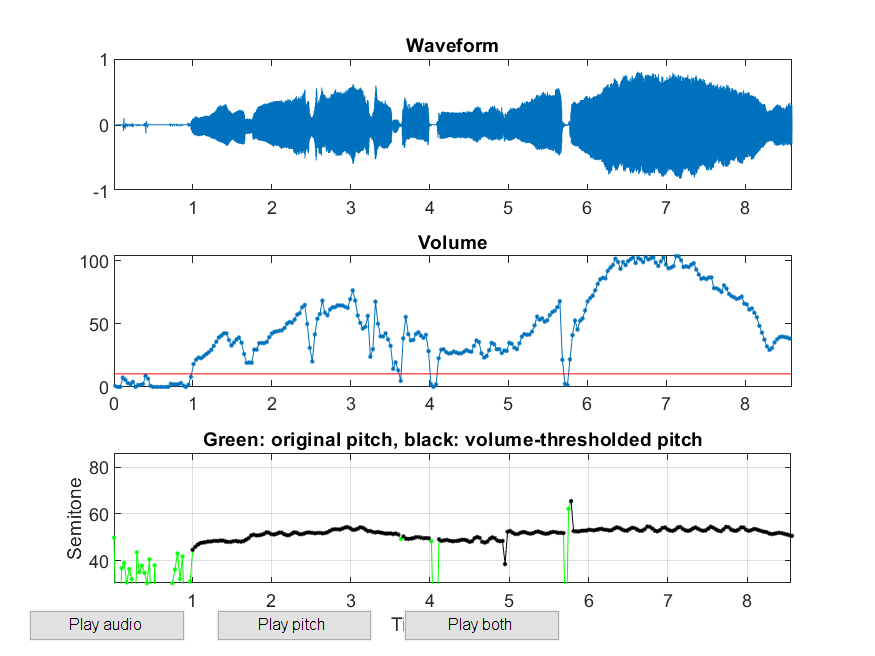There are several variations of ACF that are also used commonly:

1. The previously defined ACF has a tapering effect since a larger $\tau$ will use a smaller overlap for the calculation. As an alternative, we can compute a new ACF by dividing the inner product by the size of the overlapped region, as shown in the next equation: $$acf(\tau)=\sum_{i=0}^{n-1-\tau}\frac{s(i)s(i+\tau)}{n-\tau}$$

Due to the smaller overlap, the last few points of ACF may be unstable, as shown in the following example.

Example 5: frame2acf02.mwaveFile='sunday.wav'; au=myAudioRead(waveFile); y=au.signal; index1=9000; frameSize=512; index2=index1+frameSize-1; frame=au.signal(index1:index2); opt=frame2pdf('defaultOpt'); opt.pdf='acf'; opt.maxShift=length(frame); opt.method=2; showPlot=1; acf=frame2pdf(frame, opt, showPlot);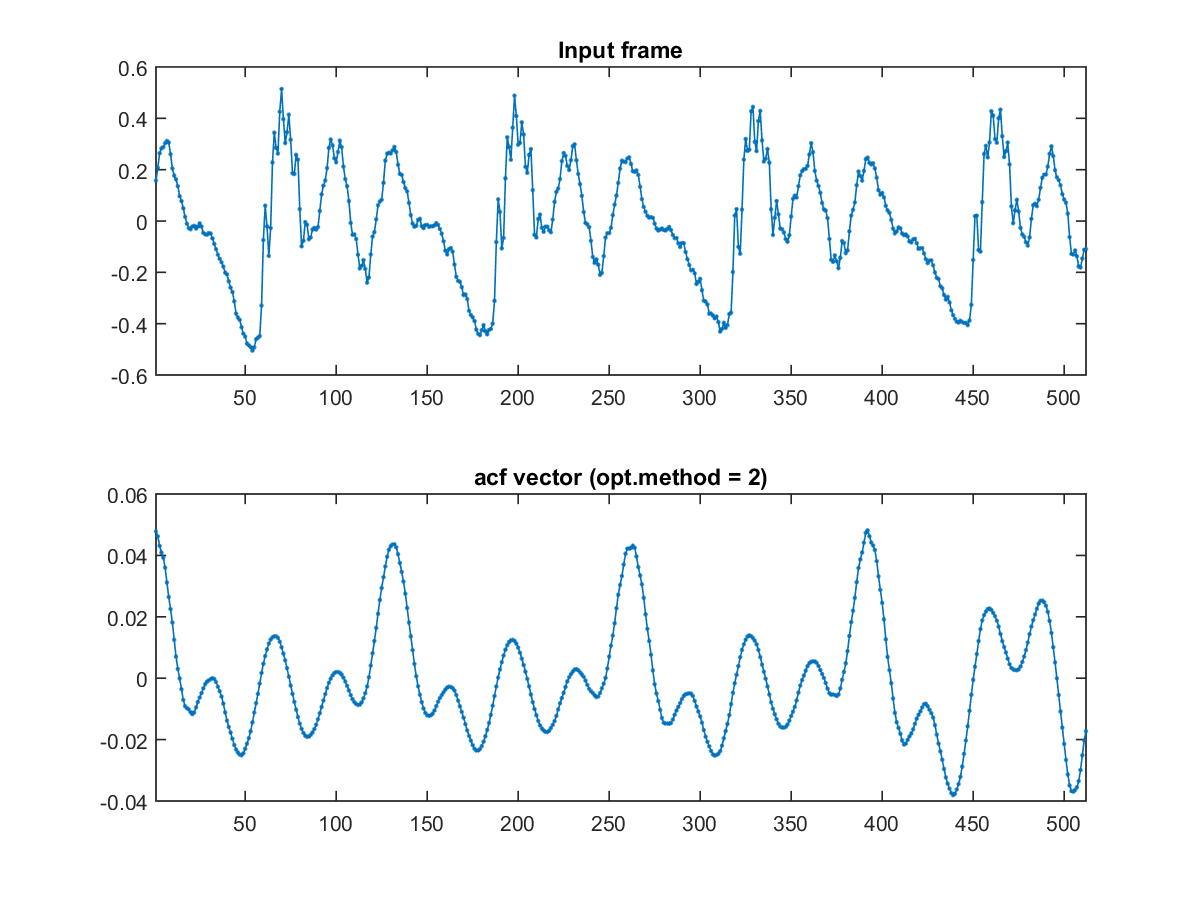The unstable ACF is reflected in the corresponding pitch tracking:

Example 6: ptByAcf02.mwaveFile='soo.wav'; opt=pitchTrackBasic('defaultOpt'); opt.frame2pitchOpt.method=2; showPlot=1; pitch=pitchTrackBasic(waveFile, opt, showPlot);2. Another method to avoid tapering-off is to shift only the first half of a frame, as shown in the next equation: $$acf(\tau)=\sum_{i=0}^{n/2}s(i)s(i+\tau)$$ The overlap region will always be half of the frame size and the obtain ACF will not taper off. Please refer to the following example.

Example 7: frame2acf03.mwaveFile='sunday.wav'; au=myAudioRead(waveFile); index1=9000; frameSize=512; index2=index1+frameSize-1; frame=au.signal(index1:index2); opt=frame2pdf('defaultOpt'); opt.pdf='acf'; opt.maxShift=length(frame)/2; opt.method=3; showPlot=1; acf=frame2pdf(frame, opt, showPlot);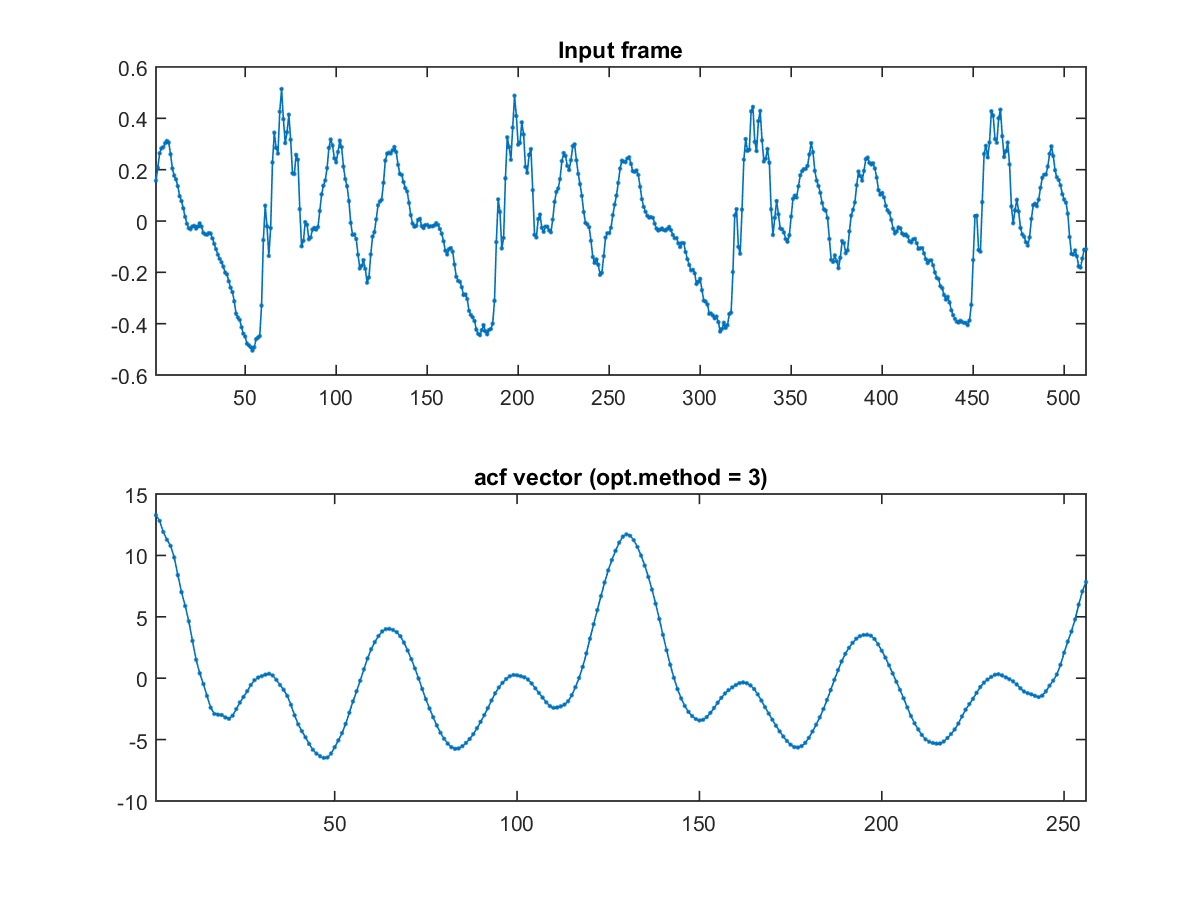However, the resultant ACF has a length of half of the frame size. Now you run into the risk of not being able to detect low pitch less than 16000/256 = 62.5 Hz. To avoid this, you might want to double the frame size (at the cost of reducing time resolution), as shown next:

Example 8: ptByacf03.mwaveFile='soo.wav'; opt=pitchTrackBasic('defaultOpt'); opt.frameDuration=32*2; % Duration (in ms) of a frame opt.overlapDuration=32; % Duration (in ms) of overlap opt.frame2pitchOpt.method=3; showPlot=1; pitch=pitchTrackBasic(waveFile, opt, showPlot);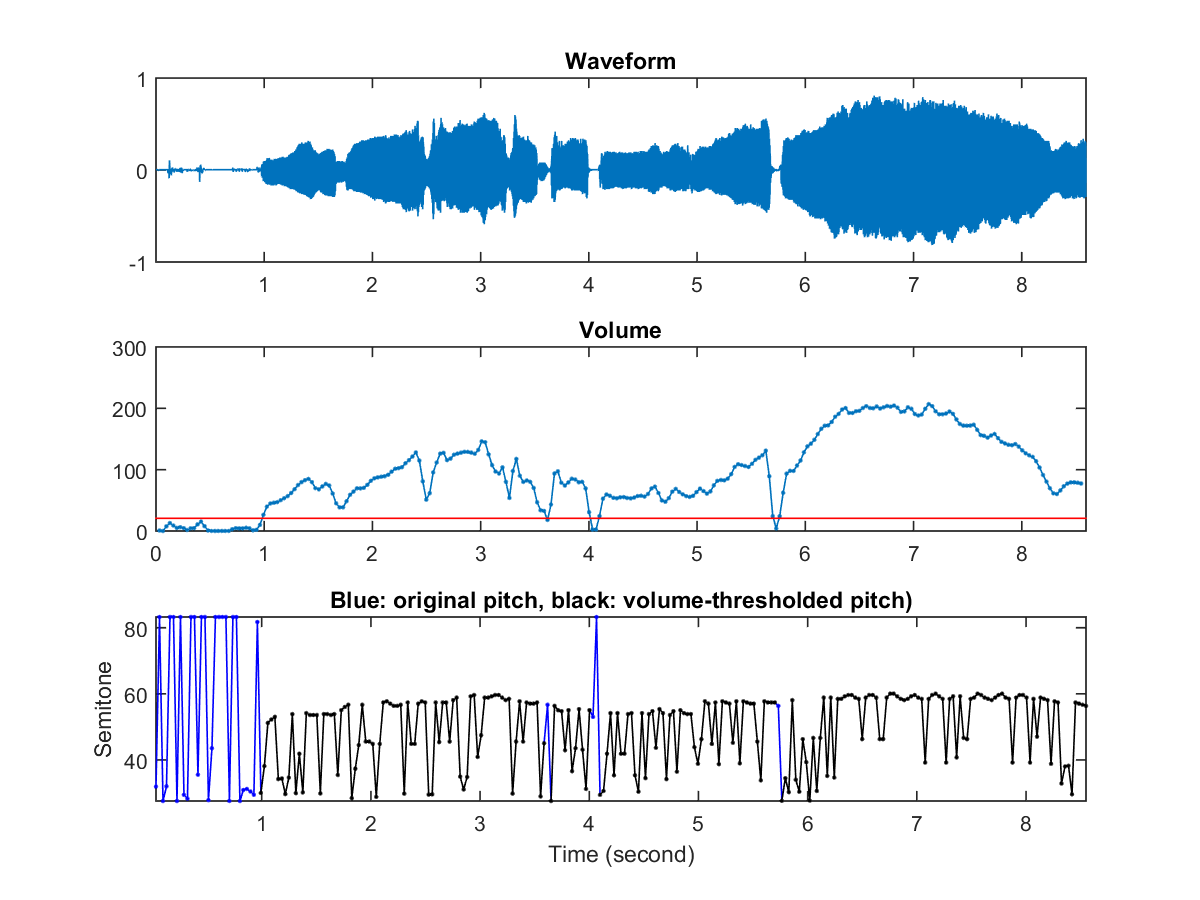Audio Signal Processing and Recognition (音訊處理與辨識)